# HC Verma Solutions Class 11 Chapter 11 Gravitation

HC Verma Class 11 Solutions Chapter 11 Gravitation has a collection of accurate solutions for questions that are found in class 11 HC Verma textbook. Some of the questions in this chapter are related to various topics such as finding the gravitational force of attraction and linear momentum of objects when the gravitational force is acting upon them and much more. With the solutions provided, students will get to learn topics of gravitation in problems such as:

• Finding the potential energy of objects when they are separated.
• Finding the direction of the field and displacement of the particle.

Additionally, we will be seeing questions related to gravity on Mount Everest and more. However, the solutions are given for every question and it will help students to learn the right method to solve these questions and also in a better way. Making use of the solutions will not only help students in gaining conceptual clarity but it will also help them to prepare effectively for JEE Main and Advanced . Students can clear all their doubts with respect to the topics discussed in the class 11 physics syllabus here.

Practising the problems given in solutions of HC Verma will significantly help students enhance their problem-solving skills.

Some of the topics that students will come across and study in chapter 11 are as follows;

1. Historical Introduction
2. Measurement of Gravitational Constant G
3. Gravitational Potential Energy
4. Gravitational Potential
5. Calculation of Gravitational Potential
6. Gravitational Field
7. The relation between Gravitational Field and Potential
8. Calculation of Gravitational Field
9. Variation in the Value of g
10. Planets and Satellites
11. Kepler’s Laws
12. Weightlessness in a Satellite
13. Escape Velocity
14. Gravitational Binding Energy
15. Black Holes
16. Inertial and Gravitational Mass
17. Possible Changes in the Law of Gravitation

## Class 11 Important Questions in Chapter 11

1. When a spacecraft travels from the earth to the moon it consumes more fuel than coming back from the moon. Explain this phenomenon.

2. An object will have greater weight at the poles than at the equator. Do you think is it profitable to purchase goods at the equator and sell them at the pole? Do you think that using a spring balance or an equal-beam balance is necessary to be used?

3. Suppose the radius of the earth decreases by 1 per cent but there is no change in its mass. What will happen to the acceleration due to gravity at the surface of the earth? Will it increase or decrease and by what per cent?

4. Let’s bring a mass of 1 kg from infinity to a point A. Initially, the mass was at rest but it then moves at a speed of 2 m/s to reach point A. The work done on the mass is 3 J. Determine the potential at A.

(a) —5 J/kg (b) —2 J/kg (c) —3 J/kg (d) none of these.

5. If we consider the magnitude of the gravitational potential energy of the moon-earth system as U with zero potential energy at infinite separation and the kinetic energy of the moon with respect to the earth is K. Choose the right option;

(a) U = K. (b) U > K. (c) U < K.

## HC Verma Solutions Vol 1 Gravitation Chapter 11

Question 1: The spherical balls of mass 10 kg each are paced 10 cm apart. Find the gravitational force of attraction between them.

Solution:

The mass of each ball = m = 10 kg

Distance of separation = r = 10 cm or 0.010 cm

Force = GMm/r2 = [6.67×10-11x102]/(0.010) 2

= 6.67 x 10-7

Question 2: Four particles having masses m, 2m, 3m and 4m are placed at the four corners of a square of edge α. Find the gravitational force acting on a particle of mass m placed at the center.

Solution: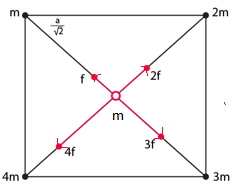The gravitational force at the center = vector sum of all the forces acting on it.

The distance between the center particle with others, say r = a/√2

Force acting between particles of mass m and center particle = Fm = GMm/r2 = 2Gm2/a2

Force acting between particles of mass m and center particle = F2m = GM(2m)/r2 = (4Gm^2)/r2 = 2Fm

Similarly, we can calculate of mass 3m and 4m along with the center particle:

F3m =3 Fm and F4m = 4 Fm

The net force:

Fnet = 2 Fm cosθ = 4 Fm (1/√2) = 2√2 Fm

=> Fnet = 2√2 Fm = 2√2 x 2Gm2/a2 = [4√2 Gm2]/a2

Question 3: Three equal masses m are placed at the three corners of an equilateral triangle of side α. Find the force exerted by the system on another particle of mass m placed at

(a) The mid-point of a side,

(b) At the Centre of the triangle.

Solution:

(a)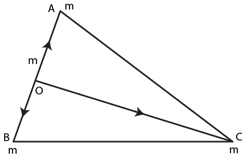If “m” is the mid point of a side, then

FOA = 4Gm2/a2 in OA direction

FOB = 4Gm2/a2 in OB direction

=> FOC = 4Gm2/3a2 in OC direction

[As, Equal and opposite cancel each other]

So, net gravitational force on m is 4Gm2/a2

(b)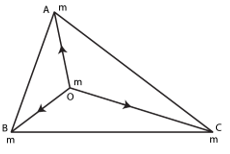If point “O” is the centroid, then

FOA = 3Gm2/a2 and FOB = 3Gm2/a2

So, resultant force is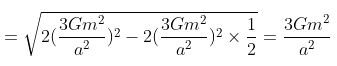Since FOC = 3Gm2/a2

[Equal and opposite to F cancel each other]

=> Net gravitational force become zero.

Question 4: Three uniform spheres each having a mass M and radius α are kept in such a way that each touches the other two. Find the magnitude of the gravitational force on any of the spheres due to the other two.

Solution:

Distance between the centers of two spheres = r= 2a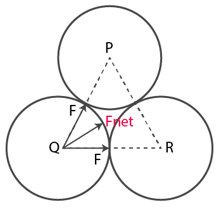Force on one sphere due to another = F = GM2/4a2

Net force = Fnet = 2F cosθ = 2F cos 30o

= 2 x √3/2 x GM2/4a2

=> Fnet = √3GM2/4a2

Question 5: Four particle of equal masses M move along a circle of radius R under the action of their mutual gravitational attraction. Find the speed of each particle.

Solution:

Let A, B, C and D are four particles of mass , moving in a circle of radius R.

Force between A and B = FAB = GM2/(√2R) 2 = GM2/2R2

Force between A and D = FAD = GM2/(√2R) 2 = GM2/2R2 = FAB

Net force in downward direction = FD = 2FAB cos 45o = √2 FAE

Force between A and C = FAC = GM2/(2R) 2 = GM2/4R2

Now,

Net force on particle A = Fnet = FD + FAC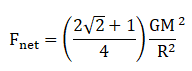For moving along the circle, F_net = mv2/R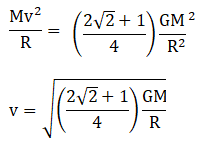Question 6: Find the acceleration due to gravity of the moon at a point 1000 Km above the moon’s surface. The mass of the moon is 7.4 × 1022 kg and its radius is 1740 km.

Solution:

Mass of the moon= M = 7.4× 1022 kg

Radius =R = 1740 km and Distance of the point from surface= R’ = 1000 km

Total distance from the center = r = 1740+1000 = 2740 km

Now,

Find Acceleration due to gravity:

g = GM/r2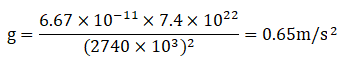Question 7: Two small bodies of masses 10 kg and 20 kg are kept a distance of 1.0 m apart and released. Assuming that only mutual gravitational forces are acting, find the speed of particles when the separation decreases to 0.5 m.

Solution:

let m1 and m2 masses of bodies, where m1 = 10 kg and m2 = 20 kg

Initial separation, say r1 = 10 m and Final separation, say r2 = 0.5 m

Let v1 be the initial velocity and v2 be the final velocity, where v1 = v2 = 0 m/s

Let us consider v1‘, v2‘ are the final velocities.

Now,

m1 v1’ + m2 v2’ = 0

=> v1’ = -(20/10)v2’ = – 2 v2

[From momentum conservation]

Again, from using the conservation of energy:

PEinitial + KEinitial = PEfinal + KEfinal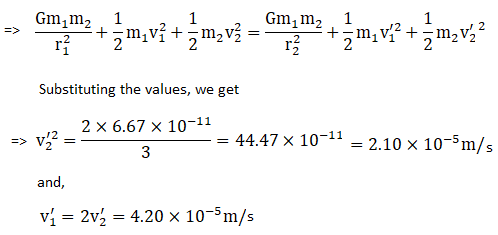Question 8: A semicircular wire has a length L and mass M. A particle of mass m is placed at the Centre of the circle. Find the gravitational attraction on the particle due to the wire.

Solution:

Let us take a small element on the wire. The arc length of the element is r dθ .

=> Mass of the element = dM = (M/L) r dθ

Also, r = L/π

=> dM = Mdθ/π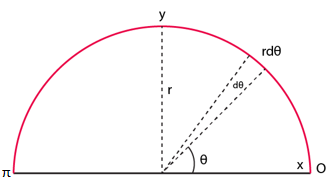The force on particle due to element = dF = GmdM/r2= (GMmπdθ)/L2

Therefore,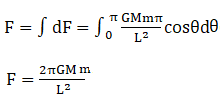Question 9: Derive an expression for the gravitational field due to a uniform rod of length L and mass M at a point on its perpendicular bisector at a distance d from the center.

Solution:

A small section of rod is at “x” distance mass of the element = dm = (M/L).dx

dE1 = [G(dm)]/(d2+x2) = dE2

So resultant dE = 2 dE1 sinθ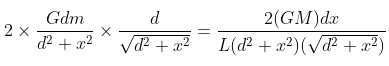Now, the total gravitational force: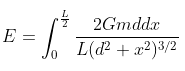Solving above equation, we get

E = 2GM/[d √(L2+4d2)]

Question 10: Two concentric spherical shells have masses M1, M2 and radii R1, R2 (R1<R2). What is the force exerted by this system on a particle of mass m1 if it is placed at a distance (R1+ R2)/2 from the center?

Solution:

The gravitational force on m due to shell of M2 is zero. Ma is at distance (R1 + R2)/2

The gravitational force: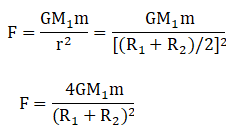Question 11: A tunnel is dug along a diameter of the earth. Find the force on a particle of mass m placed in the tunnel at a distance x from the center.

Solution:

Let us assume that tunnel doesn’t change the gravitational field distribution of earth.

Mass of the sphere: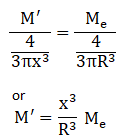Where Me is the mass of the earth .

The gravitational force on the particle at distance x,

F = GMM’/x2 = GMe/R3

Question 12: A tunnel is dug along a chord of the earth at a perpendicular distance R/2 from the earth’s center. The well of the tunnel may be assumed to be frictionless. Find the force exerted by the wall on a particle of mass m when it is at a distance x from the center of the tunnel.

Solution:

Let ME be the mass of the earth.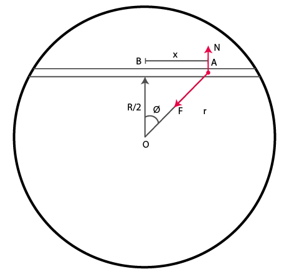From figure,

N = F cos φ

here, cos φ = R/2r and F = GME mr/R3

thus, N = GME mr/R3 x R/2r

or N = GMm/2R2

Question 13: A solid sphere of mass m and radius r is placed inside a hollow thin spherical shell of mass M and radius R as shown in figure (below). A particle of mass m’ is placed on the line joining the two centers at a distance x from the point of contact of the sphere and the shell. Find the magnitude of the resultant gravitational on this particle due to the sphere and the shell if (a) r < x < 2r, (b) 2r < x < 2R. and (C) x > 2R.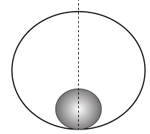Solution:

(a) distance of the particle from the center of solid sphere:

I = x – r

Gravitational force on the object:

F = Gmm’/r3

Here, the mass of the sphere “m” and m’ is the place at distance x from O.

=> F = Gmm’(x-r)/r3

(b) 2r < x < 2R, then F is due to only sphere

F = Gmm’/(x-r)^2

(c) If x > 2R, the gravitational force is due to both shell and sphere,

Force due to shell: F = GMm’/(x-R)2

Force due to sphere: F = GMm’/(x-r)2

So, resultant force = GMm’/(x-R)2 + GMm’/(x-r)2

Question 14: A uniform metal sphere of radius α and mass M is surrounded by a thin uniform spherical shell of equal mass and radius 4α (figure below). The Centre of the shell falls on the surface of the inner sphere. Find the gravitational field at the points P1 and P2 shown in the figure.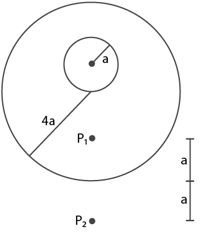Solution:

At point P1

Gravitational force due to sphere = M = GM/(3a+a)2 = GM/16a2

At point P2,Gravitational force due to sphere and shell

= GM/(a+4a+a) 2 + GM/(4a+a) 2

= (61/900) GM/a2

Question 15: A thin spherical shell having uniform density is cut in two parts by a plane and kept separated as shown in figure (below). The point A is the Centre of the plane section of the first part and B is the Centre of the place section of the second part. Show that the gravitational field at A due to the first part is equal in magnitude to the gravitational field at B due to the second part.Solution:

we know, the field inside the shell is zero. Let the gravitational field at A due to the first part be E and the gravitational field at B due to the second part be E’.

Therefore, E + E’ = 0

or E = -E’

Hence, the fields are equal is magnitude and opposite in direction

Question 16: Two small bodies of masses 2.00 kg and 4.00 Kg are kept at rest at a separation of 2.0 m. Where a particle of mass 0.10 kg should be placed to experience no net gravitational force from these bodies? The particle is placed at this point. What is the gravitational potential energy of the system of three particles with usual reference level?

Solution:

Let the mass of 0.10 kg be at a distance x from 2 kg mass and at the distance of (2-x) from the 4 kg mass.

Force between 0.1 kg mass and 4 kg mass = Force between 0.1 kg mass and 2 kg mass

(2×0.1)/x2 = -(4×0.1)/(2-x) 2

x = 2/2.414

or x = 0.83 m from the 2 kg mass.

Now,

The gravitational potential energy is given by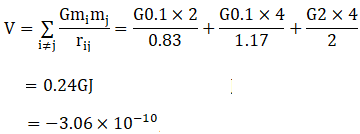Question 17: Three particles of mass m each are placed at the three corners of an equilateral triangle of side α. Find the work which should be done on this system to increase the sides of the triangle to 2α.

Solution:

work done = W = Uf – Ui

Where Uf = Final potential energy and Ui = Initial potential energy

Here, Uf = -3Gm2/2a and Ui = -3Gm2/a

Now, W = 3Gm2/2a

Question 18: A particle of mass 100 g is kept on the surface of a uniform sphere of mass 10 kg and radius 10 cm. Find the work to be done against the gravitational force between them to take the particle away from the sphere.

Solution:

Uf = Final potential energy = 0

[As the particle is to be taken away, we assume the final point to be approximately at infinite distance]

and Ui = Initial potential energy = (-GMs m)/r

Here, m = Mass of the particle = 100 g or 0.1 kg

Ms = Mass of sphere = 10 kg

And r = radius of sphere = 10 cm or 0.1 m

On putting values, we get

Ui = -6.67 x 10-10

Now, work done = W = -(Uf – Ui)

=> W = 6.67 x 10-10 J

Question 19: The gravitational field in a region is given by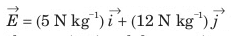(a)Find the magnitude of the gravitational force acting on a particle of mass 2 kg placed at the origin.

(b)Find the potential at the points (12m, 0) and (0, 5m) if the potential at the origin is taken to be zero.

(c) Find the change in gravitational Potential energy if a particle of mass 2 kg is taken from the origin to the point (12m, 5m).

(d)Find the change in potential energy If the particle is taken from (12m, 0) to (0, 5m).

Solution:

(a) force on the particle

F = mE = 2[5i+12j] = 10i + 24j N

[given mass of the particle = m = 2 kg]

Magnitude of F = 26 N

(b) Potential at (12, 0):

V = -E.r = -12i [5i+12j] = -60 J/kg

Potential at (0, 5):

V = -E.r = -5i [5i+12j] = -60 J/kg

(c) potential energy at (12,5) m:

V = [5i+12j] [2i + 5j] = -120 J/kg

And potential energy at the origin is zero.

Therefore, the change in potential energy is -240 J.

(d) Change in potential energy = 0

[from part (b), potential energy of the particle would be same at both the points.]

Question 20: The gravitational field in a region is given by V= 20N Kg-1 (x+y).

(a) Show that the equation is dimensionally correct.

(b) Find the gravitational field at the point (x, y). Leave your answer in terms of the unit vectors i, j, k

(c) Calculate the magnitude of the gravitational force on a particle of mass 500g placed at the origin.

Solution:

(a) V= 20N Kg-1 (x+y)

Dimension of V = [MLT-2]/M x L = L2T-2

Dimension of j/kg = [ML2T-2]/M = L2T-2

Hence dimensions are correct.

(b)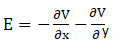Or E = -20i – 20j N/kg

E is independent of the coordinate.

(c) Force = mE

= 0.5 x [-20(i+j)]

= -10i – 10j

Magnitude of Force = 10√2 N

Question 21: The gravitational field in a region is given by E = (2i + 3j)Nkg-1. Show that no work is done by the gravitational field whom a particle is moved on the line 3y +2x = 5.

[Hint: if a line y=mx + c makes angle θ with the X – axis, m= tan θ]

Solution:

Electric field = E = 2i + 3j

Angle made by E with the x-axis: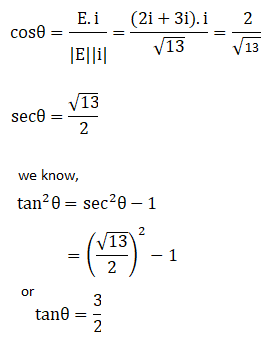Equation of line is y = -(2/3)x + 5/3 made angle with x-axis is

tanφ = -2/3

Now,

tan(φ – θ) = [tanφ – tanθ]/[1+tanφ tanθ]

= infinity

Now, the angle between the electric filed and the line = φ – θ = 90o

Since product of both the slope is -1, the direction of field and the displacement are perpendicular, is done by the particle on the line.

Question 22: Find the height over the earth’s surface at which the weight of a body becomes half of its value at the surface.

Solution:

Let h be the height

Therefore, (1/2) Gm/R2 = GM/(R+h) 2

or 2R2 = (R+h) 2

or h = (√2 – 1)R

Question 23: What is the acceleration due to gravity on the top of Mount Everest? Mount Everest is the highest mountain peak of the world at the height of 8848 m. The value at sea level is 9.80 ms-2.

Solution:

Height of Mount Everest = h = 8848 m or 8.848 km

Acceleration due to gravity at a height h, say g’

g’ = g(1 – 2h/R)

= 9.8(1 – 640/(6400×103)

= 9.799 m/s2

Question 24: Find the acceleration due to gravity in a mine of depth 640 m if the value at the surface is 9.800 ms-2. The radius of the earth is 6400 Km.

Solution:

Let g’ be the acceleration due to gravity

g’ = g (1 – 2h/R)

= 98(1-0.64/6400)

= 9.799 m/s2

Question 25: A body is weighed by a spring balance to be 1.000 kg at the North Pole. How much will it weigh at the equator? Accounts for the earth’s rotation only.

Solution:

Let g’ be the acceleration due to gravity at equation and that of pole g

Angular velocity of earth = ω = 2π/T

Now, acceleration due to gravity at equator:

g’ = g – ω2 R

= 9.8 – [2π/(24×3600)] 2 x 64000

= 9.767 m/s2

And the weight at equator = mg’ = 1 x 9.767 N = 0.997 kg

Question 26: A body stretches a spring by a particular length at the earth’s surface at equator. At what height above the South Pole will it stretch the same spring by the same length? Assume the earth to be spherical.

Solution:

Acceleration due to gravity at equator = g’ = g- ω2 R

Acceleration due to gravity at a height above south pole = g” = g(1-2h/R)

Now g’ = g”

=> g- ω2 R = g(1-2h/R)

=> h = ω2 R2/2g

Or h = [4 π x 64000002]/[(4×3600)2x2x9.8]

= 10 km (approx)

Question 27: At what rate should the earth rotate so that the apparent g at the equator becomes zero? What will be the length of the day in this situation?

Solution:

for apparent g at equator be zero.

g’ = g – ω2 R = 0

or g = ω2 R

=> ω = √(g/R) = √(9.8/6400000)

Now, T = 2 π / ω

= [2×3.14]/[1.237×10-3x3600]

= 1.4 h (approx.)

Question 28: A pendulum having a bob of mass m is happening in a ship sailing along the equator from east to west. When the ship is stationary with respect to water the tension in the string is T0.

(a) Find the speed of the ship due to rotation of the earth about its axis.

(b) Find the difference between T0 and the earth’s attraction on the bob.

(c) If the ship sails at speed v. what is the tension in the string? Angular speed of earth’s rotation is ω and radius of the earth is R.

Solution:

a) the speed of the ship is equal to earth’s rotation when the ship is stationary point.

speed = ωR

(b) tension in the string at the equator

T0 = mg’ = mg – mω2R

mg – T0 = mω2R

Difference between T0 and the earth’s attraction on the bob.

(c) angular speed of the ship is v/R about its center.

Total angular speed = ω’ = ω – v/R

And T = mg – mω’2R [tension given]

=> T = mg – m(ω – V/R)2 R

=> T = mg – [mω2 + mv2/R2 – 2mωv/R)R]

=> T = mg – mω2 R – mv2/R + 2mωv

From part (b),

T0 = mg’ = mg – mω2 R

=> T = T0 – mv2/R + 2mωv

=> T = T0 + 2mωv

Neglect, mv2/R, As small quantity.

Question 29: The time taken by the Mars to revolve round the sun is 1.88 years. Find the ratio of average distance between Mars and the sun to that between the earth and the sun.

Solution:

From Kepler’s third law, the time period of an orbit is proportional to the cube of the radius of the orbit.

T2 α R3

Tm2/T2 = RMS3/RSE3

RMS/RSE = (3.534)1/3 = 1.52

Question 30: The moon takes about 27.3 days to revolve round the earth in a nearly circular orbit of radius 3.84 × 105 Km. Calculate the mass of the earth from these data.

Solution:

For an orbit, the time period:

T2 = 4 π2a3/GM

[here a = 3.84 x 105 km and T = 27.3 x 24×3600 sec]

Or M = 6.02 x 1024 kg

Question 31: A Mars satellite moving in an orbit of radius 9.4 × 103 Km takes 27540 s to complete one revolution. Calculate the mass of Mars.

Solution:

For an orbit, the time period:

T2 = 4 π2a3/GM

Or M = 4 π2a3/G T2 …….(1)

Where M = mass of mars.

Here, Radius of mars=a = 9.4×103 km or 9.4 x 106 m and Time = T = 27540 s

Now, (1)=> M = [4 π2 (9.4×106)3]/[6.67×10-11x275402]

Or M = 6.5 x 1023 kg

Question 32: A satellite of mass 1000 kg is supposed to orbit the earth at a height of 2000 Km above the earth’s surface. Find

(a) Its speed in the orbit.

(b) Its kinetic energy.

(c) The potential energy of the earth-satellite system and

(d) Its time period. Mass of the earth = 6 × 1024 Kg.

Solution:

(a) Radius of the orbit = a = 2000 + 6400 = 8400 km or 8.4 x 106 m

Therefore, the speed = v = √(GM)/a

v = √[(6.67×10-11x6x1024)/(8.4×106)] = 6.9 km/s (approx.)

(b) KE = (1/2)mv2

Here m = 1000 kg (mass of satellite)

KE = (1/2) x 1000 x 69002 = 2.38 x 1010

(c) Potential energy at infinity is zero. Hence, the potential energy at a radius, a

PE = -GMm/a

= [-6.67×10-11x6x1024x1000]/[8.4×106]

= -4.76 x 1010

(d) Time period

T2 = 4 π2a3/GM

= [4 π2(8.4×106)3]/[6.67x 10-11x6x1024]

= 2.12 hours

Question 33: (a) Find the radius of the circular orbit of a satellite moving with an angular speed equal to the angular speed of earth’s rotation.

(b) If the satellite is directly above the North Pole at some instant, find the time it takes to come over the equatorial plane.

Mass of the earth = 6 × 1024 Kg.

Solution:

(a) Time period of revolution of satellite: T = 24×3600 = 86400 sec

Let “a” be the radius of the orbit.

T2 = 4 π2a3/GM

Or a3 = GM T2/4 π2

= [6.67x 10-11x6x1024 x 864002]/[ 4 π2]

= 7.56 x 1022

Or a = 42300 km (approx.)

(b) A complete revolution takes 24 hours, therefore a quarter of revolution is 24/4 = 6 hours

Question 34: What is the true weight of an object in a geostationary satellite that weighed exactly 10.0 N at the North Pole?

Solution:

Weight at north pole, Wp α 1/R2

Let h is distance of the satellite from earth.

Weight of satellite at equator, We α 1/(R+h)2

Now, ratio is Wp/ We = (R+h) 2/R2

We = [WpR2]/[(R+h)2]

We are given, Wp = 10 N and h = 36000 km [Height of the geostationary satellite,]

Therefore, We = [10×64002]/[(6400+36000)2] = 0.23 N(approx.)

Question 35: The radius of planet is R1 and a satellite revolves round it in a circle of radius R2. The time period of revolution is T. Find the acceleration due to the gravitation of the planet at its surface.

Solution:

The time period of revolution: T2 = 4 π2a3/GM

Or GM = 4 π2(R2)3/ T2

Or GM/R12 = 4 π2(R2)3/ T2 R12

Now, Acceleration due to gravity :

g = GM/ R12

or g = 4 π2(R2)3/ T2 R12

Question 36: Find the minimum colatitude which can directly receive a signal from a geostationary satellite.

Solution: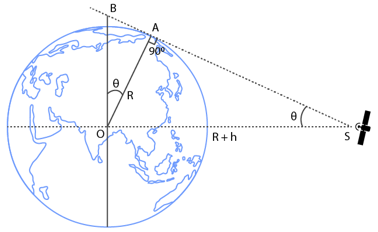form figure, angle BOA = angle OSA

In triangle AOS

sinθ = AO/OS

= R/(R+h)

= 6400/(6400+36000)

= 0.15 (approx)

or θ = sin-1(0.15)

Question 37: A particle is fired vertically upward from the earth’s surface and it goes up to a maximum height of 6400 km. Find the initial speed of the particle.

Solution:

Let KEi be initial KE and PEi initial potential energy of the system.

KEi = (1/2)mv2 and PEi = -GMm/R

KEf = 0 [at the maximum height]

And PE at height h is h = 6400 km

And PEf = -GMm/(R+h)

Now, From conservation of energy, KEi + PEi = KEf + PEf

=> (1/2)mv2 – GMm/R = -GMm/(R+h)

=> (1/2)mv2 = GMm[(-R+R+h)/R(R+h)] = GMmh/R(R+h)

Or v2 = 2GMh/R(R+h)

= [2×6.67×10-11x6x1024x6400x103]/[2x(6400×103)2]

= 7.9 km/s

Question 38: A particle is fired vertically upward with a speed of 15 km s-1. With what speed will it move in interstellar space. Assume only earth’s gravitational field.

Solution:

Let KEi be initial KE and PEi initial potential energy of the system.

KEi = (1/2)mvi2 and PEi = -GMm/R

Final KE = KEf = (1/2)mvf2

Final potential energy = PEf = 0

Using energy conservation, we have

KEi + PEi = KEf + PEf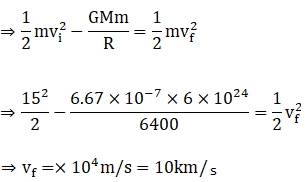Question 39: A mass of 6 × 1024 Kg (equal to the mass of the earth) is to be compressed in a sphere in such a way that the escape velocity from its surface is 3 × 108 ms-1. What should be the radius of the sphere?

Solution:

We have, (1/2) mv2 = GMm/R

or R = 2GM/v2

R = [2×6.67×10-11x6x1024]/[(3×108)2]

R = 9 mm (approx.)